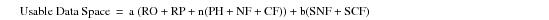# 15.00 - Field Mode Spool Space Sizing Equation - Teradata Database

prodname
vrm_release
15.00
category
User Guide
featnum
B035-1094-015K

### Field Mode Spool Space Sizing Equation

Use the following equation to determine the amount of usable data space for Field mode:The following parameter definitions are used with this equation:

 Parameter Definition a Number of rows being selected. RO Row overhead (22 bytes per row). RP Row parcel indicators; 8 bytes per row (rec start, rec end). n Number of columns being selected. PH Parcel headers (4 bytes per field). NF Formatted size of each numeric column (spaces, dollar signs, and commas should be included). CF Formatted size of each character column. For example, if a selected column is defined as VARCHAR(200), it takes up 200 characters even if it contains only 3 nonblank characters. The value for CF can be less than the CREATE TABLE definition of the string only if there is a FORMAT phrase. b Number of numeric columns in the ORDER BY clause (sort key). SNF Sorted numeric fields (8 bytes each). SCF Formatted size of each character column in the ORDER BY clause. If an order operation is done on a column defined as VARCHAR(200), 200 characters are allowed, even if there are only 3 nonblank characters. The value for CF can be less than the CREATE TABLE definition of the string only if there is a FORMAT phrase. The formatted length of the character string should be rounded up to the next even value, then 2 more bytes should be added to the number.